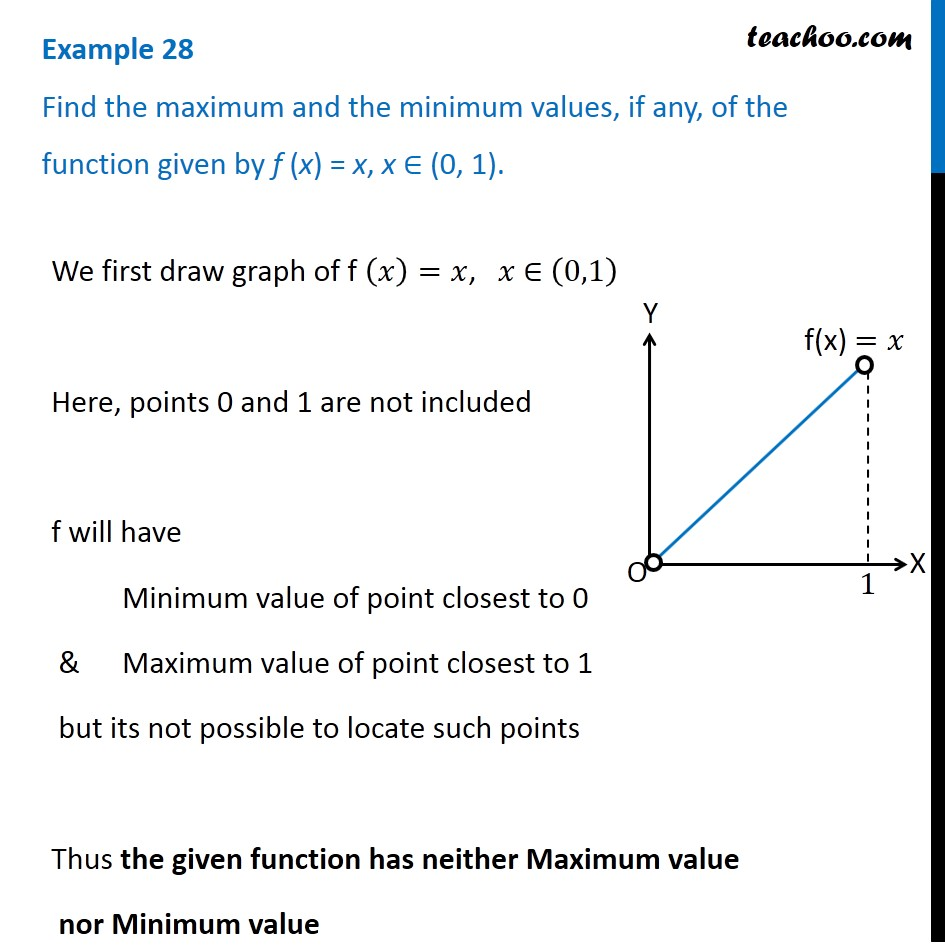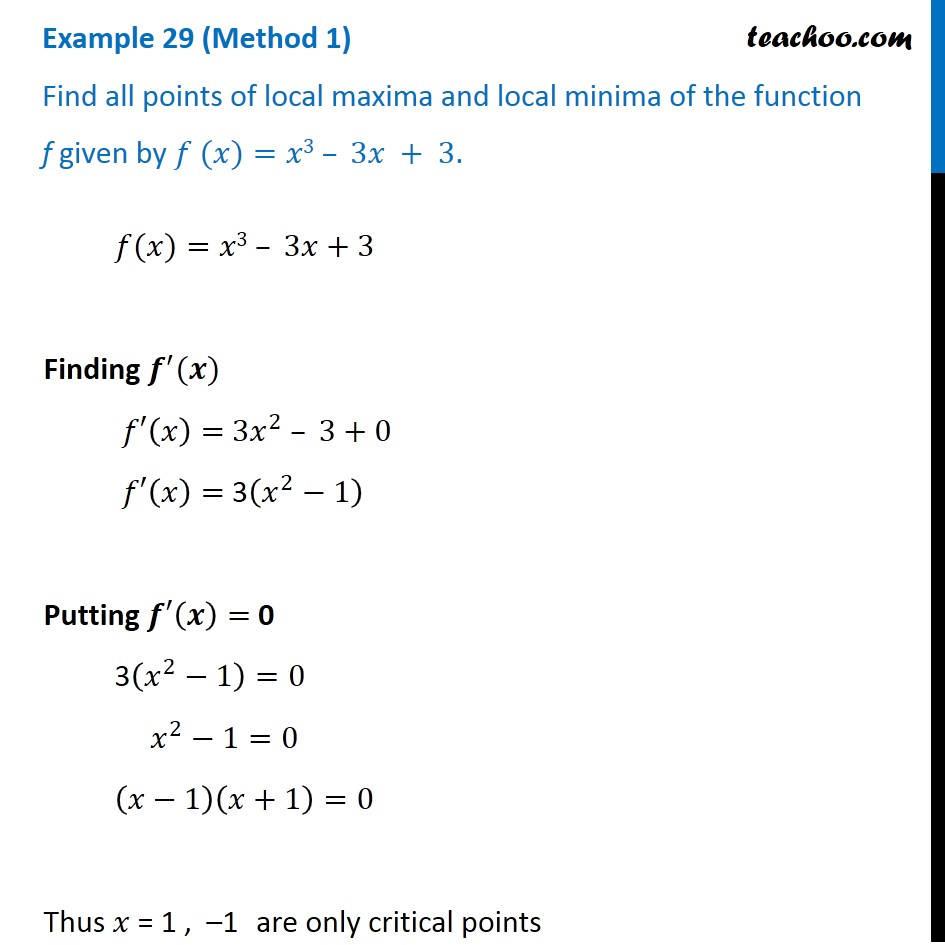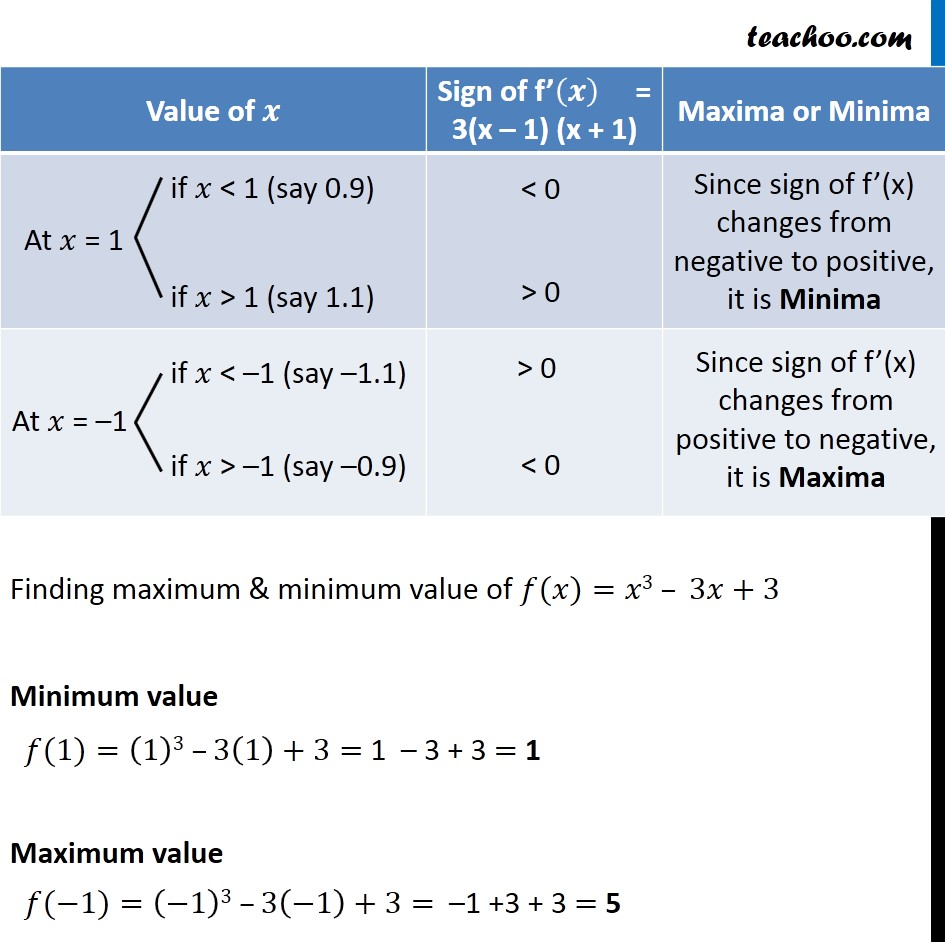Check Full Chapter Explained - Continuity and Differentiability - Application of Derivatives (AOD) Class 121. Chapter 6 Class 12 Application of Derivatives
2. Serial order wise
3. Examples

Transcript

Example 28 Find the maximum and the minimum values, if any, of the function given by f (x) = x, x ∈ (0, 1). We first draw graph of f (𝑥)=𝑥, 𝑥 ∈ (0,1) Here, points 0 and 1 are not included f will have Minimum value of point closest to 0 & Maximum value of point closest to 1 but its not possible to locate such points Thus the given function has neither Maximum value nor Minimum value

Examples